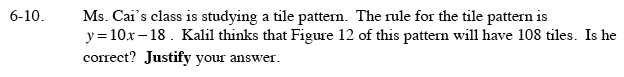### Home > CAAC > Chapter 6 > Lesson 6.1.1 > Problem6-10

6-10.Ms. Cai's class is studying a tile pattern. The rule for the tile pattern is y = 10x − 18. Kalil thinks that Figure 12 of this pattern will have 108 tiles. Is he correct? Justify your answer. Homework Help ✎y = # of tiles
x = Figure #

Substitute the information from the problem into the equation y = 10x − 18.

Kalil is wrong.

108 = 10(12) − 18
108 = 120 − 18

108 = 102 tiles